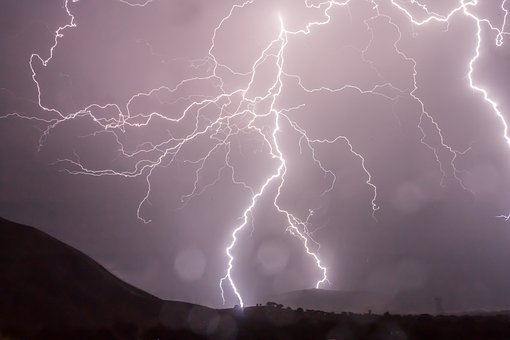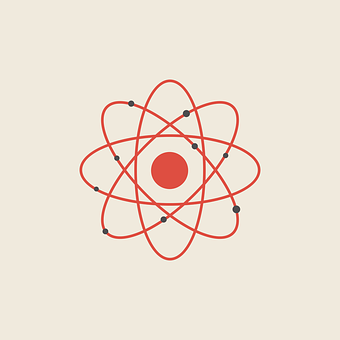# Joule Unit

## What is joule?

Joule is the SI unit of energy or work (energy and work are interconvertible). Its symbol is “J”. It is named after a Scientist James Prescott Joule who worked on the conversion of enegy which led to the law of conservation of energy.

## Equivalent units of joule:

The formula of work / energy is,

W=F.d →1

Where W stands for work, F represents force and d is the distance. We know that the unit of force is newton and in SI system distance is measured in meters so according to this, Joule is equal to N.m

Joule=Newton×meter (N.m) →2

Another equivalent unit for joule can be derived by opening the formula of force in equation 1.

F=ma

W=ma.d

Here m stands for mass and a is the acceleration. Mass is measured in kilograms and unit of acceleration is m/s2 so Joule is also equal to Kgm^2/s^2

Joule=(Kg×m×m)/s^2

Joule=(Kg.m^2)⁄s^2 →3

## Definition of joule:

Joule can be defined in many ways.

1. According to the second equation, the amount of work that is done by 1 newton of force in moving an object through a distance of 1 meter is equivalent to 1 joule.
2. According to the third equation, the amount of work that is done when 1kg of object is moved 1 meter with an acceleration of 1m/s2 is equal to 1 joule.## Joule in terms of potential difference:

Let’s see the formula of electric potential difference.

V=W/q →4

V is the potential difference and q stands for charge. The units of potential difference and charge are volt and coulomb (C). This gives us another equivalent unit of joule that is,

Joule=Volt×Coulomb### Definition of joule:

The above equation has given us another definition of joule. The amount of work done on 1 coulomb of charge when there is potential difference of 1 volt. is equal to 1 joule.

## Joule in terms of power:

P=W/t

P is power here and t is for time. Let’s look in to the units. The SI unit of power is watt and time is measured in seconds so Joule is also equivalent to power x time

Joule=watt×sec

### Definition of joule:

Joule can also be that amount of work which is done in 1 second with power of 1 watt.

### Joule into Kilowatt-hour:

Commercially energy is measured in Kilowatt-hour. 1 kilowatt-hour is equal to 36x10^5 joules.

1 Kilowatt-hour=〖10〗^3×36×〖10〗^2=36×〖10〗^5 Joules

## Conversion of joule into erg:

In CGs (centimeter gram second) system the unit of energy or work is erg.

1 erg=〖10〗^(-7) Joule

## Conversion of joule into Calorie:

Another unit of energy in CGS system is calorie.

1 cal=4.18 Joule

## Conversion of joule into dyn.cm:

Dyne (represented as dyn) is the unit of force in CGS system and in this system distance is measured in cm. According to the formula of energy / work (equation 1) in CGS another unit of energy can be dyn.cm.

1 dyn.cm=1×〖10〗^(-7) Joules

The above equation also shows that the unit dyn.cm is equal to erg.

## Conversion of joule into BTU:

BTU stands for british thermal unit. To convert joule into BTU multiply the value in joule with 1055.

1 BTU=1055 Joule

## Conversion of joule into lb.ft:

In FPS system (foot pound second) the distance is measured in foot (represented as ft) and the unit of force is pound (symbol lb). According to equation 1, in FPS system the unit of energy will be lb.ft.

1 lb.ft=1.356 Joules

## Atomic unit of Energy:

Joule and other units are not convinient to describe the energy of very small particles like electrons and protons. So scientist developed another unit to measure very small energy changes. They called it “Electron Volt”.### Definition of electron volt:

The amount of energy required by an electron to move in an electric field when the potential difference is of 1 volt.

### Conversion of joule into electron volt:

According to equation 4 (formula of potential difference),

1 ev=1.6×〖10〗^(-19) coulomb ×1 volt

1 ev=1.6×〖10〗^(-19) Joules

Energy
Watt hour
Energy conservation

James Prescott Joule (1818 − 1889) was a self-educated British physicist and brewer whose work in the mid nineteenth century contributed to the establishment of the energy concept. The international unit of energy bears
his name:
1 Joule [J] = 1 Watt-second [Ws] = 1 V A s = 1 N m = 1 kg m2s−2.
It takes about 1 J to raise a 100-g-apple 1 m. Energy units can be preceded
by various factors, including the following:
kilo (k=103), mega (M=106), giga (G=109), tera (T=1012), peta (P=1015),
Exa (E=1018).
Thus, a kiloJoule (kJ) is 1000 Joules and a mega Joule (MJ) is 1,000,000 Joules.
A related unit is the Watt, which is a unit of power (energy per unit time). Power units can be converted to energy units through multiplication by seconds [s], hours, [h], or years [yr].
For example, 1 kWh [kilowatt hour] = 3.6 MJ [mega Joule]. With 1 kWh, about 10 liters of water can be heated from 20 ºC to the boiling point.
There are many other energy units besides the “Systèm International d’Unités (SI)”. A “ton of coal equivalent” (tce) is frequently used in the energy business. 1 tce equals 8.141 MWh. It means that the combustion of 1 kg of coal produces the same amount of heat as electrical heating for one hour at a rate of 8.141 kW.
More Units of Energy:
1 calIT = 4.1868 J, International Table calorie
1 calth = 4.184 J, thermochemical calorie
1 cal15 ≈ 4.1855 J, calories to heat from 14.5 °C to 15.5 °C
1 erg = 10−7 J, cgs [centimeter-gram-second] unit
1 eV ≈ 1.60218 × 10−19 J, electron volt
1 Eh ≈ 4.35975 × 10−18 J, Hartree, atomic energy unit
1 Btu = 1055.06 J, British thermal unit according to ISO, to heat 1 pound water from 63 °F to 64 °F
1 tce = 29.3076 × 109 J, ton of coal equivalent, 7000 kcalIT
1 toe = 41.868 × 109 J, ton of oil equivalent, 10000 kcalIT
Calories and/or kilocalories [cal and/or kcal] were historically often used to measure heat (energy) and are still used fot thi sometimes today. Heating a gram of water 1 ºC requires 1 cal. Different definitions are often the result of inconsistent starting temperatures of the heating.
Multiplication Table of Units:

Symbol Exponential Prefix Quantity
k 103 kilo thousand
M 106 mega million
G 109 giga billion
T 1012 tera trillion
E 1018 exa quintillion

The unit Megagram is not used, since there is a special
name for one million grams, one ton (t): 1 t = 1000 kg.
Multiplication of the Units of Power with Units of Time:
When the Watt is multiplied by a unit of time, an energy unit is formed as follows: 1 Ws = 1 J.
The use of the kilowatt-hour is more common: 1 kWh = 3600 kWs = 3.6 MJ. Besides the second [s] and the hour [h], the day [d] and the year [yr] are also used,
with 1 yr = 365.2425 d = 31,556,952 s. So, for example, energy of one Megawatt-year can be written as 1 MWyr = 31.557952 TJ (TeraJoule).
The annual consumption of 1 toe/yr corresponds to the daily consumption of about 31.56 kWh/d.The annual consumption of 1 GJ/yr corresponds to the daily consumption of about 0.7605 kWh/d.
Conversion of Energy Units:
The unit conversions indicated on this page can be performed with a calculator. One can also find conversion calculators on the internet such as the International Energy Agency, unit conversion.or and at Robert Fogt.
Joule , unit of work or energy in the International System of Units (SI); it is equal to the work done by a force of one newton acting through one metre. Named in honour of the English physicist James Prescott Joule , it equals 107 ergs, or approximately 0.7377 foot-pounds.
The theory for electrical energy and power was developed using the principles of
mechanical energy, and the units of energy are the same for both electrical and
mechanical energy. However, heat energy is typically measured in quantities that are
separately defined from the laws of mechanics and electricity and magnetism. Sir James
Joule first studied the equivalence of these two forms of energy and found that there was
a constant of proportionality between them and this constant is therefore referred to as the
Joule equivalent of heat and given the symbol J. The Joule equivalent of heat is the
amount of mechanical or electrical energy contained in a unit of heat energy. The factor
is to be determined in this experiment.
Power is defined as the rate of doing work, and electrical power is defined as the amount
of electrical energy being expended per unit time. The work ∆W (mechanical energy)
required to move an electrical charge ∆Q through a potential difference V is given by
∆ =∆ × W QV (1)
and power is P is given by
W Qso that
PVI = × . (3)
In an electrical circuit the energy ∆W expended in a increment of time, ∆t , in a
resistance is given by
∆ = ×∆ WP t (4)
which can be written as
∆ = × ×∆ WVI t (5)
when Equation (3) is substituted for P.
Electrical and mechanical energy is measured in units of joules in the SI system of units,
but heat energy is measured in units of kilocalories.
The change in heat energy of a material, ∆Q , is directly proportional to the change in
temperature of the material and depends on the type of material and its mass. The change
is heat energy, ∆Q , for a given change of temperature, ∆T , is given by
∆= ∆ Q mc T (6)
where m is the mass of the material and c the specific heat of the material. If electrical
energy is transformed into heat energy then the equivalence of the electrical energy and
heat energy is given by
∆ = ×∆ WJ Q (7)
where J is the Joule equivalent of heat or the mechanical energy equivalent of heat
energy. In SI units, J = 4186 Joules/kilocalorie .
In this experiment a constant current I will be used to flow through a resistive heating
element and a constant potential drop V across the element will be maintained. The
electrical energy expended in the heating element will be transformed into heat energy
that is will increase the temperature of a quantity of water and the container in which it is
kept. The change in heat energy of the container and water will be the sum of the heat
energies of each and will be given by
∆ = ∆+ ∆ Q mc T m c T cc ww (8)
where mc and mw are the masses of the container and water respectively, and cc and cw are
the specific heats of the material of the container and water.
VI t J m c T m c T ×∆ = ∆ + ∆ ( cc ww ). P V